12.1 The biot-savart law  (Page 2/4)

 Page 2 / 4

Calculating magnetic fields of short current segments

A short wire of length 1.0 cm carries a current of 2.0 A in the vertical direction ( [link] ). The rest of the wire is shielded so it does not add to the magnetic field produced by the wire. Calculate the magnetic field at point P , which is 1 meter from the wire in the x -direction.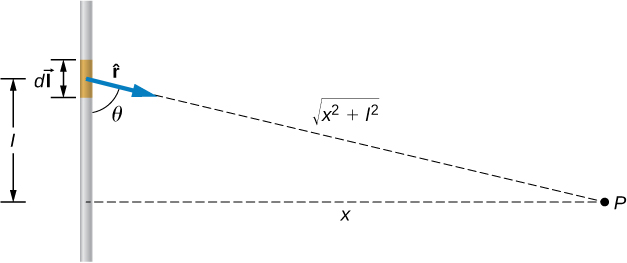A small line segment carries a current I in the vertical direction. What is the magnetic field at a distance x from the segment?

Strategy

We can determine the magnetic field at point P using the Biot-Savart law. Since the current segment is much smaller than the distance x , we can drop the integral from the expression. The integration is converted back into a summation, but only for small dl , which we now write as $\text{Δ}l.$ Another way to think about it is that each of the radius values is nearly the same, no matter where the current element is on the line segment, if $\text{Δ}l$ is small compared to x . The angle $\theta$ is calculated using a tangent function. Using the numbers given, we can calculate the magnetic field at P .

Solution

The angle between $\text{Δ}\stackrel{\to }{l}$ and $\stackrel{^}{r}$ is calculated from trigonometry, knowing the distances l and x from the problem:

$\theta ={\text{tan}}^{-1}\left(\frac{1\phantom{\rule{0.2em}{0ex}}\text{m}}{0.01\phantom{\rule{0.2em}{0ex}}\text{m}}\right)=89.4\text{°}.$

The magnetic field at point P is calculated by the Biot-Savart law:

$B=\frac{{\mu }_{0}}{4\pi }\phantom{\rule{0.2em}{0ex}}\frac{I\text{Δ}l\phantom{\rule{0.2em}{0ex}}\text{sin}\phantom{\rule{0.1em}{0ex}}\theta }{{r}^{2}}=\left(1\phantom{\rule{0.2em}{0ex}}×\phantom{\rule{0.2em}{0ex}}{10}^{-7}\text{T}\cdot \text{m/A}\right)\left(\frac{2\phantom{\rule{0.2em}{0ex}}\text{A}\left(0.01\phantom{\rule{0.2em}{0ex}}\text{m}\right)\phantom{\rule{0.1em}{0ex}}\text{sin}\left(89.4\text{°}\right)}{{\left(1\phantom{\rule{0.2em}{0ex}}\text{m}\right)}^{2}}\right)=2.0\phantom{\rule{0.2em}{0ex}}×\phantom{\rule{0.2em}{0ex}}{10}^{-9}\text{T}.$

From the right-hand rule and the Biot-Savart law, the field is directed into the page.

Significance

This approximation is only good if the length of the line segment is very small compared to the distance from the current element to the point. If not, the integral form of the Biot-Savart law must be used over the entire line segment to calculate the magnetic field.

Check Your Understanding Using [link] , at what distance would P have to be to measure a magnetic field half of the given answer?

1.41 meters

Calculating magnetic field of a circular arc of wire

A wire carries a current I in a circular arc with radius R swept through an arbitrary angle $\theta$ ( [link] ). Calculate the magnetic field at the center of this arc at point P .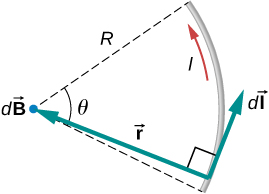A wire segment carrying a current I . The path d l → and radial direction r ^ are indicated.

Strategy

We can determine the magnetic field at point P using the Biot-Savart law. The radial and path length directions are always at a right angle, so the cross product turns into multiplication. We also know that the distance along the path dl is related to the radius times the angle $\theta$ (in radians). Then we can pull all constants out of the integration and solve for the magnetic field.

Solution

The Biot-Savart law starts with the following equation:

$\stackrel{\to }{B}=\frac{{\mu }_{0}}{4\pi }\underset{\text{wire}}{\int }\frac{Id\stackrel{\to }{l}\phantom{\rule{0.2em}{0ex}}×\phantom{\rule{0.2em}{0ex}}\stackrel{^}{r}}{{r}^{2}}.$

As we integrate along the arc, all the contributions to the magnetic field are in the same direction (out of the page), so we can work with the magnitude of the field. The cross product turns into multiplication because the path dl and the radial direction are perpendicular. We can also substitute the arc length formula, $dl=rd\theta$ :

$B=\frac{{\mu }_{0}}{4\pi }\underset{\text{wire}}{\int }\frac{Ir\phantom{\rule{0.2em}{0ex}}d\theta }{{r}^{2}}.$

The current and radius can be pulled out of the integral because they are the same regardless of where we are on the path. This leaves only the integral over the angle,

$B=\frac{{\mu }_{0}I}{4\pi r}\underset{\text{wire}}{\int }d\theta .$

The angle varies on the wire from 0 to $\theta$ ; hence, the result is

$B=\frac{{\mu }_{0}I\theta }{4\pi r}.$

Significance

The direction of the magnetic field at point P is determined by the right-hand rule, as shown in the previous chapter. If there are other wires in the diagram along with the arc, and you are asked to find the net magnetic field, find each contribution from a wire or arc and add the results by superposition of vectors. Make sure to pay attention to the direction of each contribution. Also note that in a symmetric situation, like a straight or circular wire, contributions from opposite sides of point P cancel each other.

Check Your Understanding The wire loop forms a full circle of radius R and current I . What is the magnitude of the magnetic field at the center?

$\frac{{\mu }_{0}I}{2R}$

Summary

• The magnetic field created by a current-carrying wire is found by the Biot-Savart law.
• The current element $Id\stackrel{\to }{l}$ produces a magnetic field a distance r away.

Conceptual questions

For calculating magnetic fields, what are the advantages and disadvantages of the Biot-Savart law?

Biot-Savart law’s advantage is that it works with any magnetic field produced by a current loop. The disadvantage is that it can take a long time.

Describe the magnetic field due to the current in two wires connected to the two terminals of a source of emf and twisted tightly around each other.

How can you decide if a wire is infinite?

If you were to go to the start of a line segment and calculate the angle $\theta$ to be approximately $0\text{°}$ , the wire can be considered infinite. This judgment is based also on the precision you need in the result.

Identical currents are carried in two circular loops; however, one loop has twice the diameter as the other loop. Compare the magnetic fields created by the loops at the center of each loop.

Problems

A 10-A current flows through the wire shown. What is the magnitude of the magnetic field due to a 0.5-mm segment of wire as measured at (a) point A and (b) point B?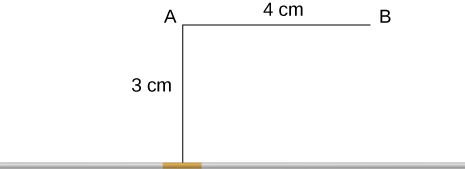Ten amps flow through a square loop where each side is 20 cm in length. At each corner of the loop is a 0.01-cm segment that connects the longer wires as shown. Calculate the magnitude of the magnetic field at the center of the loop.$1\phantom{\rule{0.2em}{0ex}}×\phantom{\rule{0.2em}{0ex}}{10}^{-8}\text{T}$

What is the magnetic field at P due to the current I in the wire shown?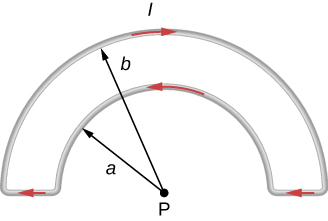The accompanying figure shows a current loop consisting of two concentric circular arcs and two perpendicular radial lines. Determine the magnetic field at point P.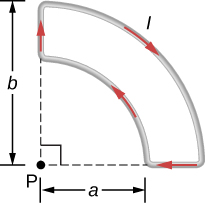$B=\frac{{\mu }_{o}I}{8}\left(\frac{1}{a}-\phantom{\rule{0.05em}{0ex}}\frac{1}{b}\right)$ out of the page

Find the magnetic field at the center C of the rectangular loop of wire shown in the accompanying figure.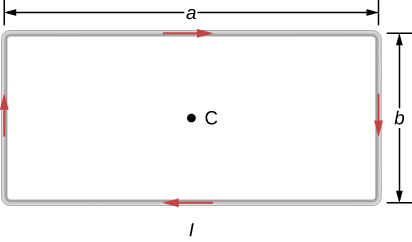Two long wires, one of which has a semicircular bend of radius R , are positioned as shown in the accompanying figure. If both wires carry a current I , how far apart must their parallel sections be so that the net magnetic field at P is zero? Does the current in the straight wire flow up or down?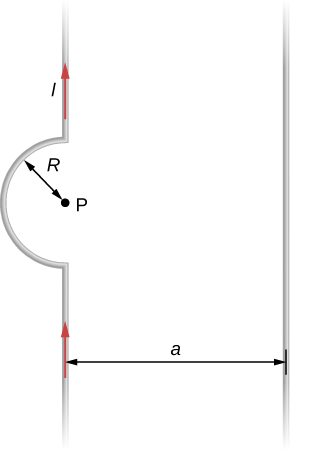$a=\frac{2R}{\pi }$ ; the current in the wire to the right must flow up the page.

in the 2nd example, for chapter 8.2 on page 3/3, I don't understand where the value 48uC comes from, I just couldn't get that value in my calculator.
what is electromagnetic force. do electric and magnetic force happen differently
yes
yes
Pranay
why
Godson
how?
Godson
derived the electric potential due to disk of charge
how can we derived potential electric due to the disk
aron
how can you derived electric potential of a disk
aron
how can you derived electric potential due to disk
aron
where is response?
aron
what is difference between heat and temperature?
temperature is the measure of degree of hotness or coldness. on the other hand, heat is the form of energy, which causes temperature. So we can safely say, heat is the reason and temperature is its consequence.
SABYASACHI
how many liquid metals do we have
do we have gasses as metals
Jeffery
who knows should please tell us
yes...gallium & cesium
Idris
Hg is liquid. No metal gasses at standard temp and pressure
Shane
I don't ever understand any of this formulae
which formula
How to determine a temperature scale
what is the formula for absolute error
Nyro
define kelvin planck statement
using p-v diagram, explain what takes place during each four processes of a carnot cycle
Masia
using p-v diagram, explain what takes place during each four processes of a carnot cycle
Masia
state zeroths law of thermodynamics
The zeroth law of thermodynamics states that if twothermodynamic systems are each in thermal equilibrium with a third one, then they are in thermal equilibrium with each other
Seza
zeroth law of thermodynamic state that when a body A and B are in thermal equilibrium to body C ,a state is attained when body A,B and C are in thermal equilibrium.
akanbi
sorry guys I got no any idea on the law can someone help me please?
SNAHR
hi
Muhsin
The zeroth law of thermodynamics states that if two thermodynamic systems are each in thermal equilibrium with a third one, then they are in thermal equilibrium with each other. Accordingly, thermal equilibrium between systems is a transitive relation. Two systems are said to be in the relation of
Muhsin
I can help u Snahr as possible
Vinayaka
it simply states that, if two bodies are separately in thermal equilibrium with a third body, then those two bodies will also be in thermal equilibrium with each other
Vinayaka
What mass of steam of 100 degree celcius must be mixed with 150g of ice at 0 degree celcius, in a thermally insulated container, to produce liquid water at 50 degree celcius
sorry I dont know
Bamidele
thank you
Emmanuel
What is the pressure?
SHREESH
To convert 0°C ice to 0°c water. Q=M*s=150g*334J/g=50100 J.......... Now 0° water to 50° water... Q=M*s*dt=150g*4.186J/g*50= 31395 J....... Which adds upto 81495 J..... This is amount of heat the steam has to carry. 81495= M *s=M*2230J/g..therefore.....M=36.54g of steam
SHREESH
This is at 1 atm
SHREESH
If there is change in pressure u can refer to the steam table ....
SHREESH
instrument for measuring highest temperature of a body is?
Thermometer
Umar
how does beryllium decay occur
Photon?
Umar
state the first law of thermodynamics
Its state that "energy can neither be created nor destroyed but can be transformed from one form to another. "
Ayodamola
what about the other laws can anyone here help with it please
Sandy
The second law of thermodynamics states that the entropy of any isolated system always increases. The third law of thermodynamics states that the entropy of a system approaches a constant value as the temperature approaches absolute zero.
sahil
The first law is very simple to understand by its equation. The law states that "total energy in thermodynamic sytem is always constant" i.e d¶=du+dw where d¶=total heat du=internal energy dw=workdone... PLEASE REFER TO THE BOOKS FOR MORE UNDERSTANDING OF THE CONCEPT.
Elia
what is distance.?
what is physics?
Ali
Physics is a scientific phenomenon that deals with matter and its properties
Ayodamola
physics is the study of nature and science
John
Chater1to7
min
Physics is branch of science which deals with the study of matters in relation with energy.
Elia
What is differential form of Gauss's law?By